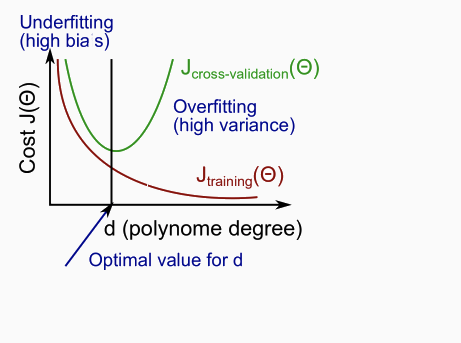If your machine learning algorithm is not working well, you can try following things.

• Get more training examples.
• Try smaller set of features.
• Decreasing or increasing λ - the regularization parameter.
##### Machine learning diagnostic.

A test you can run to gain insight what is/isn’t working with a learning algorithm, and gain guidance as to how best to improve its performance.

##### Evaluating hypothesis.
• Overfitting
• Use part of training examples as test set.
• Typically random 30% of training examples are used as test set.
• If hypothesis overfits - then error in training set will be low, but high in test set.
• Degree of polynomial
• Keep 20% of training examples for cross validation set.
• 60% training set, 20% cross validation set, 20% test set.
##### High bias or variance problem.
• Bias problem - training set and cross validation set errors both will be high.
• Variance problem - training set error is low, but cross validation errors are high.##### Learning Curve.
• Plot average squared error for training set size.
• Plot both average squared error for cross validation set (JCV</sup>) and training set Jtrain.
• As the training set gets larger, the error for a quadratic function increases.
• The error value will plateau out after a certain m, or training set size.
• High bias - JCV</sup> and Jtrain both will be high, will converge for sufficiently large value of training set size. More data will not help.
• High variance - Jtrain « JCV</sup>, will not converge. More data will likely help.
##### Neural networks.
• Smaller networks prone to under fitting.
• Larger networks prone to over fitting, but can be solved using regularization.
• Number of hidden layers is similar to degree of polynomial and same techniques can be used to optimize that.
##### Machine Learning System Design
• How to spend your time to reduce error of your algorithm?
• Collect lots of data.
• Develop sophisticated features.
• Error analysis
• Plot learning curves to decide whether you need more features or data.
• Eye ball data where algorithm is going wrong.
• Use a single numerical evaluation metric for your algorithm - error rate or something like that.
• This evaluation will helps with deciding whether your changes are useful or not.
##### Skewed classes in classification algorithm.
• Data may be skewed to contain far more number of examples for certain class.
• For example - far more samples (99%) of patients who dont have cancer, compared to small sample of patient who do.
• Throwing out data to reducing skew is incorrect, as skew is representative of real world.
• Using error metric will not be very useful to determine performance of such algorithm.
• In classification, you can change threshold from 0.5 to higher or lower values to force your algorithm to predict positive class only when very confident.
• Precision(P) - true positives / (true positives + false positives)
• What percent of predicted positive results were correct.
• Good algorithm will have closer to 1.
• Recall(R) - true positives / (true positives + false negative)
• What percent of positive results were predicted correctly
• Good algorithm will have closer to 1.
• Trade off between Precision and Recall is a hard and depends on context.
• Trying out different algorithms and comparing P and R for them is a good to determine which algorithm is better.
• F score - 2 * (P * R)/(P + R) - Higher the value the better.
• This score is used to compare performance of various algorithm.
##### Data for machine learning
• Very large data set makes even an “inferior algorithm” work better, almost at par with better algorithms.
• Although having large data set is not sufficient.
• Is the data useful and contains necessary information to write a good enough algorithm?
• Can a human expert predict output confidently based on example and set of features?
• For example - you can’t predict housing price based on data which contains only size of the house as information, irrespective of how many examples you have.